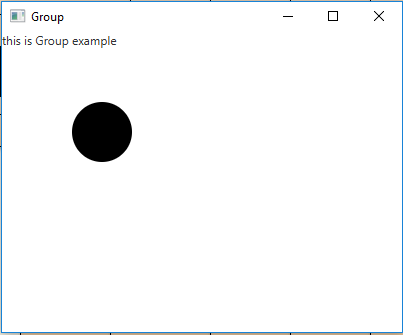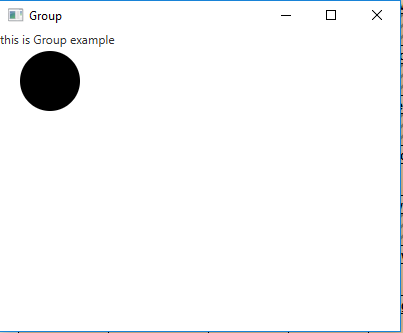# JavaFX | Group Class

Group class is a part of JavaFX. A Group contains the number of nodes. A Group will take on the collective bounds of its children and is not directly resizable. Group class inherits Parent class.

Constructor of the class:

1. Group(): Constructs a new group.
2. Group(Collection children): Constructs a new group with specified nodes.
3. Group(Node… c): Constructs a new group with specified nodes.

Commonly Used Methods:

Method Explanation
getChildren() Returns the children of the group.
isAutoSizeChildren() Gets the value of the property autoSizeChildren.
minHeight(double width) Returns the node’s minimum height for use in layout calculations.
minWidth(double height) Returns the node’s minimum width for use in layout calculations.
prefHeight(double width) Group defines the preferred height as simply being the height of its layout bounds.
prefWidth(double height) Group defines the preferred width as simply being the width of its layout bounds.
setAutoSizeChildren(boolean v) Sets the value of the property autoSizeChildren.

Below programs illustrate the use of Group class:

1. Java Program to create a Group and add it to the stage: In this program we are creating a Label named label, and a Circle named circle. Now create a Group name group and add the label and circle to it by using the getChildren().add() function. Create a scene and add the group to the scene. Add the scene to the stage and display the stage to view the final results.

 `// Java Program to create a Group ` `// and add it to the stage ` `import` `javafx.application.Application; ` `import` `javafx.scene.Scene; ` `import` `javafx.scene.control.*; ` `import` `javafx.scene.layout.*; ` `import` `javafx.stage.Stage; ` `import` `javafx.event.ActionEvent; ` `import` `javafx.event.EventHandler; ` `import` `javafx.scene.canvas.*; ` `import` `javafx.scene.web.*; ` `import` `javafx.scene.Group; ` `import` `javafx.scene.shape.*; ` ` `  `public` `class` `Group_1 ``extends` `Application { ` ` `  `    ``// launch the application ` `    ``public` `void` `start(Stage stage) ` `    ``{ ` ` `  `        ``try` `{ ` ` `  `            ``// set title for the stage ` `            ``stage.setTitle(``"Group"``); ` ` `  `            ``// create a Group ` `            ``Group group = ``new` `Group(); ` ` `  `            ``// create a label ` `            ``Label label = ``new` `Label(``"this is Group example"``); ` ` `  `            ``// add label to group ` `            ``group.getChildren().add(label); ` ` `  `            ``// circle ` `            ``Circle c = ``new` `Circle(``100``, ``100``, ``30``); ` ` `  `            ``// add Circle to Group ` `            ``group.getChildren().add(c); ` ` `  `            ``// create a scene ` `            ``Scene scene = ``new` `Scene(group, ``400``, ``300``); ` ` `  `            ``// set the scene ` `            ``stage.setScene(scene); ` ` `  `            ``stage.show(); ` `        ``} ` ` `  `        ``catch` `(Exception e) { ` ` `  `            ``System.out.println(e.getMessage()); ` `        ``} ` `    ``} ` ` `  `    ``// Main Method ` `    ``public` `static` `void` `main(String args[]) ` `    ``{ ` ` `  `        ``// launch the application ` `        ``launch(args); ` `    ``} ` `} `

Output:2. Java Program to create a Group, set auto resize to true and add it to the stage: In this program we are creating a Label named label and a Circle named circle. Then we will create a Group name group and add the label and circle to it by using the getChildren().add() function. Set the auto size children to true using the setAutoSize() function. Create a scene and add the group to the scene. Add the scene to the stage and display the stage to view the final results.

 `// Java Program to create a Group, ` `// set auto resize to true ` `// and add it to the stage ` `import` `javafx.application.Application; ` `import` `javafx.scene.Scene; ` `import` `javafx.scene.control.*; ` `import` `javafx.scene.layout.*; ` `import` `javafx.stage.Stage; ` `import` `javafx.event.ActionEvent; ` `import` `javafx.event.EventHandler; ` `import` `javafx.scene.canvas.*; ` `import` `javafx.scene.web.*; ` `import` `javafx.scene.Group; ` `import` `javafx.scene.shape.*; ` ` `  `public` `class` `Group_2 ``extends` `Application { ` ` `  `    ``// launch the application ` `    ``public` `void` `start(Stage stage) ` `    ``{ ` ` `  `        ``try` `{ ` ` `  `            ``// set title for the stage ` `            ``stage.setTitle(``"Group"``); ` ` `  `            ``// create a Group ` `            ``Group group = ``new` `Group(); ` ` `  `            ``// create a label ` `            ``Label label = ``new` `Label(``"this is Group example"``); ` ` `  `            ``// add label to group ` `            ``group.getChildren().add(label); ` ` `  `            ``// circle ` `            ``Circle c = ``new` `Circle(``50``, ``50``, ``30``); ` ` `  `            ``// set auto resize ` `            ``group.setAutoSizeChildren(``true``); ` ` `  `            ``// add Circle to Group ` `            ``group.getChildren().add(c); ` ` `  `            ``// create a scene ` `            ``Scene scene = ``new` `Scene(group, ``400``, ``300``); ` ` `  `            ``// set the scene ` `            ``stage.setScene(scene); ` ` `  `            ``stage.show(); ` `        ``} ` ` `  `        ``catch` `(Exception e) { ` ` `  `            ``System.out.println(e.getMessage()); ` `        ``} ` `    ``} ` ` `  `    ``// Main Method ` `    ``public` `static` `void` `main(String args[]) ` `    ``{ ` ` `  `        ``// launch the application ` `        ``launch(args); ` `    ``} ` `} `

Output:Note: The above programs might not run in an online IDE. Please use an offline compiler.My Personal Notes arrow_drop_upCheck out this Author's contributed articles.

If you like GeeksforGeeks and would like to contribute, you can also write an article using contribute.geeksforgeeks.org or mail your article to contribute@geeksforgeeks.org. See your article appearing on the GeeksforGeeks main page and help other Geeks.

Please Improve this article if you find anything incorrect by clicking on the "Improve Article" button below.

Article Tags :
Practice Tags :

Be the First to upvote.

Please write to us at contribute@geeksforgeeks.org to report any issue with the above content.